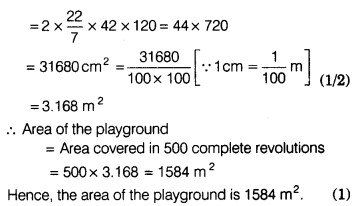# The diameter of a roller is 84 cm and its length is 120 cm. It takes 500 complete

The diameter of a roller is 84 cm and its length is 120 cm. It takes 500 complete revolutions to move once over to level a playground. Find the area (in m2) of the playground.[Take π =22/7]

Given, diameter of a roller, d = 84 cm
Radius of a roller, r = d/2 = 84/2 = 42 cm
Length of roller, h = 120 cm
∴ Area covered in 1 revolution
= Curved surface area of the roller
= 2πrh [∵ roller is in cylindrical shape]